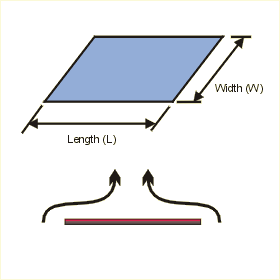Close

This page calculates the average heat transfer coefficient and plate temperature for an isothermal (constant temperature) heated horizontal flat plate's upper surface or a cooled plate's lower surface in a natural convection environment. The convection calculation automatically switches between calculation methods based on Rayleigh number.The calculation is based on Nusselt number correlations.

The heat flow (q) from the plate is calculated as:

q = h A x (Tp - Ta)

Where h is the average heat transfer coefficient, A is the area of the plate, Tp is the plate temperature and Ta is the ambient fluid temperature. h is defined as:

h = Nu k / cl

Where Nu is the Nusselt Number, k the conductivity of the fluid and L the length of the plate. The Nusselt number is calculated as:

For Ra values between 2x104 and 106
Nu = 0.54 (Gr* Pr)0.25

For Ra values between 106 and 1011
Nu = 0.15 (Gr* Pr)0.33

For the above equations Ra is the Rayleigh number, Gr is the Grashof number, and Pr is the Prandtl number and are calculated using fluid properties as follows:

Ra = (Gr * Pr)
Pr = kinematic viscosity / thermal diffusivity
Gr = gravity x (coefficient of expansion) x (Tp - Ta) x cl3 / (kinematic viscosity)2

Where cl is the characteristic dimension which is calculated using:

cl = area / perimeter

In addition, you must define the fluid properties at the film temperature Tf defined as follows, except for the density which is defined at the reference temperature of 20 C.

Tf = (Tp + Ta) / 2

The fluid density at the film temperature is automatically calculated from the following relationship based on the perfect gas law:

Rho = Rhoref (Tref +273) / (Tf + 273)

References

Lloyd, J. R. and W. R. Moran, Natural Convection Adjacent to Horizontal surface of Various Planforms, ASME Pap. 74-WA/HT-66.

Fujii, T. and H. Imura, Natural Convection Heat Transfer from a Plate with Arbitrary Inclination, Int. J. Heat Mass Transfer, vol. 15, p. 755, 1972.

Holman, J.P., Heat Transfer, 7th ed., McGraw Hill Book Company, New York, 1990. p. 333 - 352